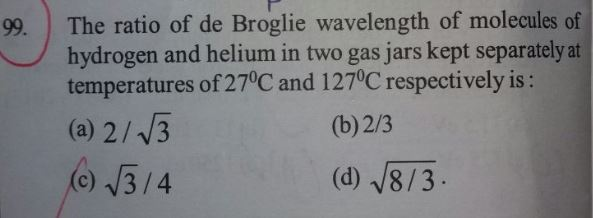# The ratio of the de-Broglie wavelength of molecule o   of hydrogen and helium atom in two gas jar kept separetely at temperature of 27oC and 127oC respectively is(A)  2 / 3½    (B)    2/3     (C)     3½ /4      (D) (8/3)½5 years ago
Dear Faiz

We know that, de-Broglie wavelength λ = h/mv.

where the speed (r.m.s) of a gas particle at the given temperature (T) is given as,

½ mv2 = 3/2 KT

or, v = √ 3kT/m

Here K is Boltzmann’s constant, m is mass of the gas particle and T is the temperature of gas in K.

So, mv = √3mKT

or, λ = h/mv = h/√3mKT

Thus, λHHe = √ mHeTHe/mHeTH = √ (4 amu) (273+127º)K / (2 amu) (273+27º)K = √8/3

Regards